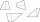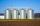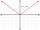Trapezium internal angles

A trapezium where AB is parallel to CD, has angle A : angle D = 4 :5, angle B = 3x-15 and angle C = 4x+20. Find angle A, B, C and D.

Result

A =  80 °
B =  60 °
C =  120 °
D =  100 °

Solution:

A = 4/5 D
B = 3x-15
C = 4x+20
A+D = 180
B+C = 180

5A-4D = 0
B-3x = -15
C-4x = 20
A+D = 180
B+C = 180

A = 80
B = 60
C = 120
D = 100
x = 25

Calculated by our linear equations calculator.

Leave us a comment of example and its solution (i.e. if it is still somewhat unclear...):

Showing 1 comment:Partho
Let Angle A=4x, B= 3x-15, C=4x+20 and D=5x.

Using the property that sum of adjacent angles = 180 we get:

A + D = 180 or 4x+5x=180.
Solving we get x=20.
Substituting we get: A=80, B=100

Similarly, B+C=180 or
3x-15+4x+20=180
Solving we get x=25
Substituting we get: B=60, D=120.To solve this example are needed these knowledge from mathematics:

Do you have a linear equation or system of equations and looking for its solution? Or do you have quadratic equation? See also our trigonometric triangle calculator.

Next similar examples:

1. Internal angles ISTDetermine internal angles of isosceles trapezium ABCD /a, c are the bases/ and if: alpha:gamma = 1:3
2. AngleDetermine the size of the smallest internal angle of a right triangle which angles forming the successive members of the arithmetic sequence.
3. Alfa beta gamaThe triangle's an interior angle beta is 10 degrees greater than the angle alpha and gamma angle is three times larger than the beta. Determine the size of the interior angles.
4. Angles in ratioThe size of the angles of the triangle are in ratio x: y = 7: 5 and the angle z is 42° lower than the angle y. Find size of the angles x, y, z.
5. In a 2In a thirteen sided polygon, the sum of five angles is 1274°, four of the eight angles remaining are equal and the other four are 18° less than each of the equal angles. Find the angles. .
6. Angles of the triangleABC is a triangle. The size of the angles alpha, beta are in a ratio 4: 7. The angle gamma is greater than the angle alpha by a quarter of a straight angle. Determine angles of the triangle ABC.
7. A triangleA triangle has an angle that is 63.1 other 2 are in ratio of 2:5 What are the measurements of the two angles?
8. Isosceles trapezoidPerimeter of the isosceles trapezoid is 48 cm. One side is two times greater than the second side. Determine the dimensions of the trapezoid.
9. Largest angle of the triangleWhat is the largest angle of the triangle if the second angle is 10° greater than twice the first and the third is 30° smaller than the second?
10. Linsys2Solve two equations with two unknowns: 400x+120y=147.2 350x+200y=144
11. Ball gameRichard, Denis and Denise together scored 932 goals. Denis scored 4 goals over Denise but Denis scored 24 goals less than Richard. Determine the number of goals for each player.
12. ChildrenThe group has 42 children. There are 4 more boys than girls. How many boys and girls are in the group?
13. Geometric sequence 5About members of geometric sequence we know: ? ? Calculate a1 (first member) and q (common ratio or q-coefficient)
14. Men, women and childrenOn the trip went men, women and children in the ratio 2:3:5 by bus. Children pay 60 crowns and adults 150. How many women were on the bus when a bus was paid 4,200 crowns?
15. WarehousesIn the three warehouses, a total of 70 tons of grain was stored. In the second warehouse was stored 8.5t less and in the third 3.5t more than in the first. How many tons of grain was stored in each warehouse?
16. Null pointsCalculate the roots of the equation: ?
17. Theorem proveWe want to prove the sentence: If the natural number n is divisible by six, then n is divisible by three. From what assumption we started?